Junction Field Effect Transistor - Semiconductor Devices, CSIR-NET Physical Sciences

# Junction Field Effect Transistor - Semiconductor Devices, CSIR-NET Physical Sciences Notes | Study Physics for IIT JAM, UGC - NET, CSIR NET - Physics

## Document Description: Junction Field Effect Transistor - Semiconductor Devices, CSIR-NET Physical Sciences for Physics 2022 is part of Physics for IIT JAM, UGC - NET, CSIR NET preparation. The notes and questions for Junction Field Effect Transistor - Semiconductor Devices, CSIR-NET Physical Sciences have been prepared according to the Physics exam syllabus. Information about Junction Field Effect Transistor - Semiconductor Devices, CSIR-NET Physical Sciences covers topics like and Junction Field Effect Transistor - Semiconductor Devices, CSIR-NET Physical Sciences Example, for Physics 2022 Exam. Find important definitions, questions, notes, meanings, examples, exercises and tests below for Junction Field Effect Transistor - Semiconductor Devices, CSIR-NET Physical Sciences.

Introduction of Junction Field Effect Transistor - Semiconductor Devices, CSIR-NET Physical Sciences in English is available as part of our Physics for IIT JAM, UGC - NET, CSIR NET for Physics & Junction Field Effect Transistor - Semiconductor Devices, CSIR-NET Physical Sciences in Hindi for Physics for IIT JAM, UGC - NET, CSIR NET course. Download more important topics related with notes, lectures and mock test series for Physics Exam by signing up for free. Physics: Junction Field Effect Transistor - Semiconductor Devices, CSIR-NET Physical Sciences Notes | Study Physics for IIT JAM, UGC - NET, CSIR NET - Physics
 1 Crore+ students have signed up on EduRev. Have you?
• In 1945, Shockley had an idea for making a solid state device out of semiconductors.
• He reasoned that a strong electrical field could cause the flow of electricity within a nearby semiconductor.
• He tried to build one, but it didn't work.
• Three years later, Brattain & Bardeen built the first working transistor, the germanium point-contact transistor, which was designed as the junction (sandwich) transistor.
• In 1960 Bell scientist John Atalla developed a new design based on Shockley's original field-effect theories.
• By the late 1960s, manufacturers converted from junction type integrated circuits to field effect devices.
• Field effect devices are those in which current is controlled by the action of an electron field, rather than carrier injection.
• Field-effect transistors are so named because a weak electrical signal coming in through one electrode creates an electrical field through the rest of the transistor.
• The FET was known as a “unipolar” transistor.
• The term refers to the fact that current is transported by carriers of one polarity (majority), whereas in the conventional bipolar transistor carriers of both polarities (majority and minority) are involved.

The family of FET devices may be divided into :

• Junction FET
• Depletion Mode MOSFET
• Enhancement Mode MOSFET

Junction  FETs  (JFETs)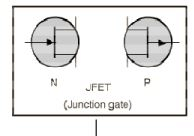•  JFETs consists of a piece of high-resistivity semiconductor material (usually Si) which constitutes a channel for the majority carrier flow.
• Conducting semiconductor channel between two ohmic contacts – source & drain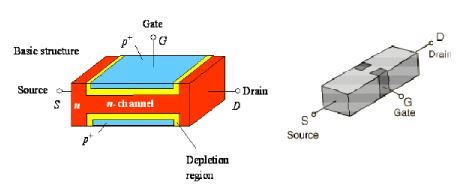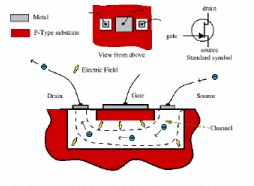• The magnitude of this current is controlled by a voltage applied to a gate, which is a reverse-biased.
• The fundamental difference between JFET and BJT devices: when the JFET junction is reverse-biased the gate current is practically zero, whereas the base current of the BJT is always some value greater than zero.

Junction  FETs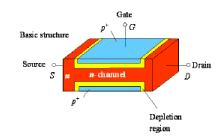• JFET is a high-input resistance device, while the BJT is comparatively low.
• If the channel is doped with a donor impurity, n-type material is formed and the channel current will consist of electrons.
• If the channel is doped with an acceptor impurity, p-type material will be formed and the channel current will consist of holes.
• N-channel devices have greater conductivity than pchannel types, since electrons have higher mobility than do holes; thus n-channel JFETs are approximately twice as efficient conductors compared to their p-channel counterparts.

Basic Structure of  FETs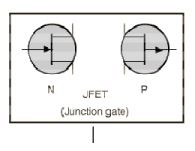• In addition to the channel, a JFET contains two ohmic contacts: the source and the drain.
• The JFET will conduct current equally well in either direction and the source and drain leads are usually interchangeable.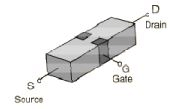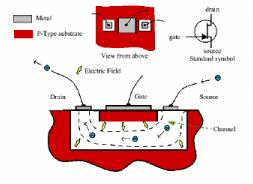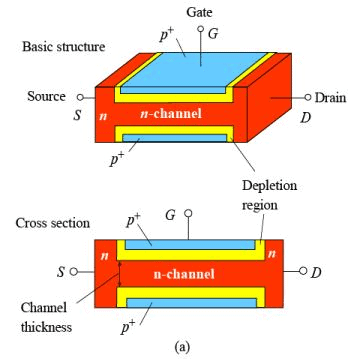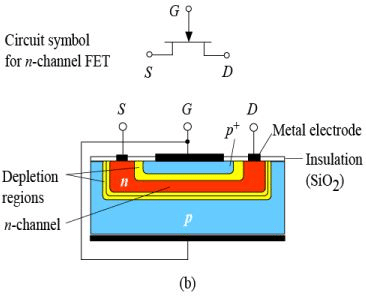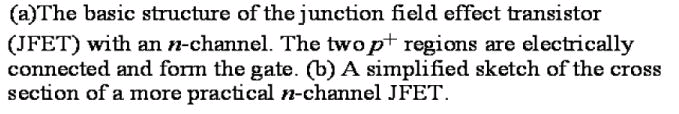N - Channel JFET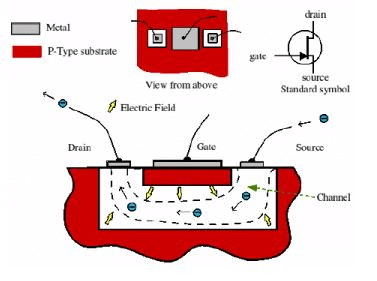• This transistor is made by forming a channel of N-type material in a P-type substrate.
• Three wires are then connected to the device.
• One at each end of the channel.
• One connected to the substrate.
• In a sense, the device is a bit like a PN-junction diode, except that there are two wires connected to the Ntype side.

How JFET  Function

• The gate is connected to the source.
• Since the pn junction is reversebiased, little current will flow in the gate connection.
• The potential gradient established will form a depletion layer, where almost all the electrons present in the n-type channel will be swept away.
• The most depleted portion is in the high field between the G and the D, and the leastdepleted area is between the G and the S.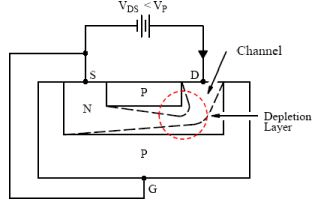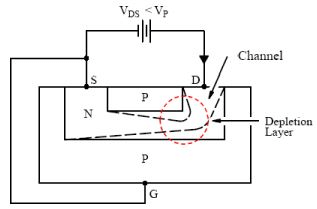• Because the flow of current along the channel from the (+ve) drain to the (-ve) source is really a flow of free electrons from S to D in the n-type Si, the magnitude of this current will fall as more Si becomes depleted of free electrons.
• There is a limit to the drain current (ID) which increased VDS can drive through the channel.
• This limiting current is known as IDSS (Drain-to-Source current with the gate shorted to the source).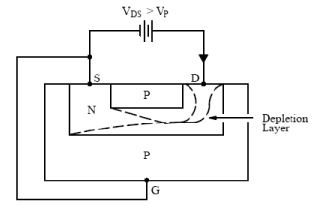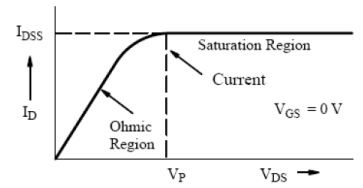• The output characteristics of an n-channel JFET with the gate short-circuited to the source.
• The initial rise in ID is related to the buildup of the depletion layer as VDS increases.
• The curve approaches the level of the limiting current IDSS when ID begins to be pinched off.
• The physical meaning of this term leads to one definition of pinch-off voltage, VP , which is the value of VDS at which the maximum IDSS flows.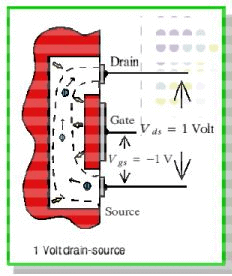• With a steady gate-source voltage of 1 V there is always 1 V across the wall of the channel at the source end.
• A drain-source voltage of 1 V means that there will be 2 V across the wall at the drain end. (The drain is ‘up’ 1V from the source potential and the gate is 1V ‘down’, hence the total difference is 2V.)
• The higher voltage difference at the drain end means that the electron channel is squeezed down a bit more at this end.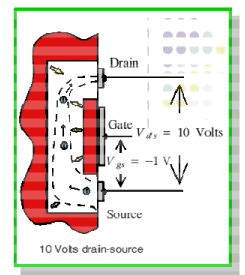•  When the drain-source voltage is increased to 10V the voltage across the channel walls at the drain end increases to 11V, but remains just 1V at the source end.
• The field across the walls near the drain end is now a lot larger than at the source end.
• As a result the channel near the drain is squeezed down quite a lot.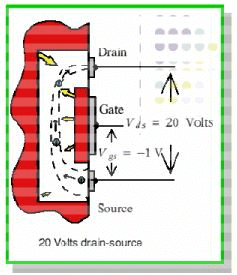•  Increasing the source-drain voltage to 20V squeezes down this end of the channel still more.
• As we increase this voltage we increase the electric field which drives electrons along the open part of the channel.
• However, also squeezes down the channel near the drain end. l This reduction in the open channel width makes it harder for electrons to pass.
• As a result the drain-source current tends to remain constant when we increase the drain-source voltage.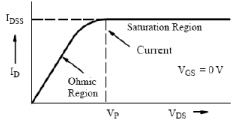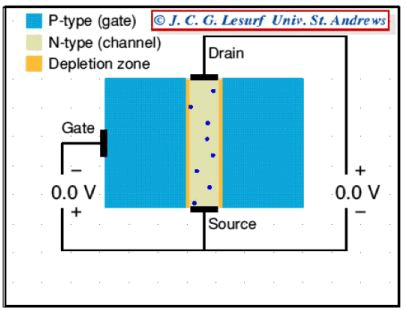•  Increasing VDS increases the widths of depletion layers, which penetrate more into channel and hence result in more channel narrowing toward the drain.
• The resistance of the n-channel, RAB therefore increases with VDS
• The drain current:  IDS = VDS/RAB
• ID versus VDS exhibits a sublinear behavior, see figure for VDS < 5V.
• The pinch-off voltage, VP is the magnitude of reverse bias needed across the p+n junction to make them just touch at the drain end.
• Since actual bias voltage across p+n junction at drain end is VGD, the pinch-off occur whenever: VGD = -VP.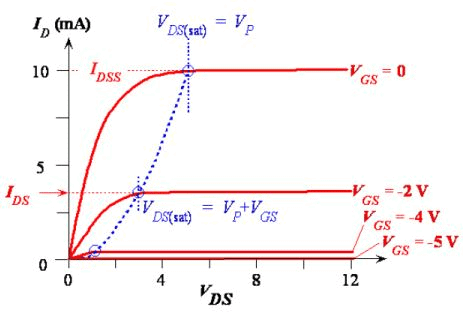Typical ID vs VDs characteristics of a JFET for various fixed gate voltages VGS.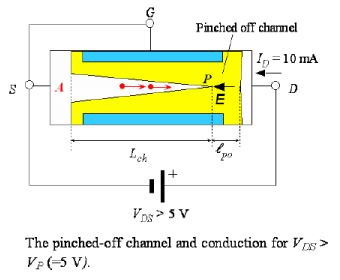•  Beyond VDS = VP, there is a short pinch-off channel of length, ℓpo.
• As VDS increases, most of additional voltage simply drops across ℓpo as this region is depleted of carriers and hence highly resistive.
• Voltage drop across channel length, Lch remain as VP.
• Beyond pinch-off then   ID = VP/RAP (VDS>VP).

• What happen when negative voltage, says VGS = -2V, is applied to gate with respect to source (with VDS=0).
• The p+n junction are now reverse biased from the start, the channel is narrower, and channel resistance is now larger than in the VGS = 0 case.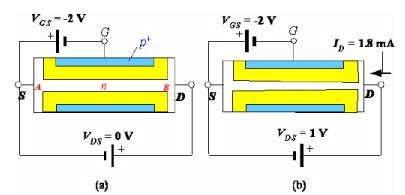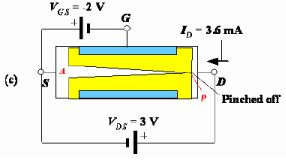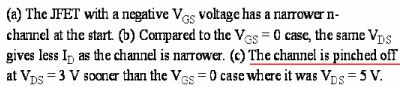• The drain current that flows when a small VDS applied (Fig b) is now smaller than in VGS= 0 case.
• Applied VDS= 3 V to pinch-off the channel (Fig c).
• When VDS= 3V, VGD across p+n junction at drain end is -5V, which is –VP, so channel becomes pinch-off.
• Beyond pinch-off, ID is nearly saturated just as in the VGS=0 case.
• Pinch-off occurs at VDS= VDS(sat),  VDS(sat)= VP+VGS, where VGS is –ve voltage (reducing VP).
• For VDS>VDS(sat), ID becomes nearly saturated at value as IDS.
• Beyond pinch-of, with –ve VGS, IDS is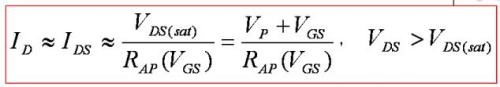• Where RAP(VGS) is the effective resistance of the conducting n-channel from A to P, which depends on channel thickness and hence VGS.
• When VGS= -VP= -5V with VDS= 0, the two depletion layers touch over the entire channel length and the whole channel is closed.
• The channel said to be off.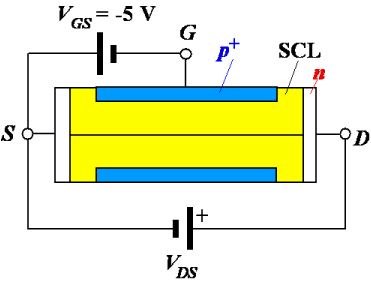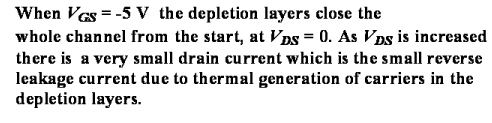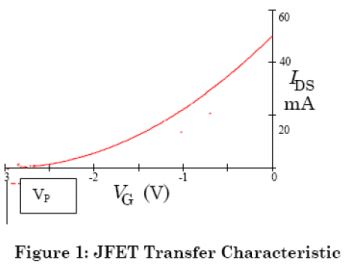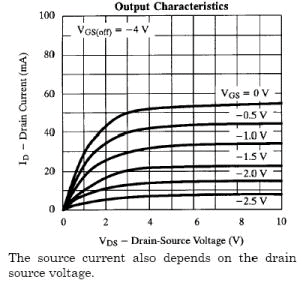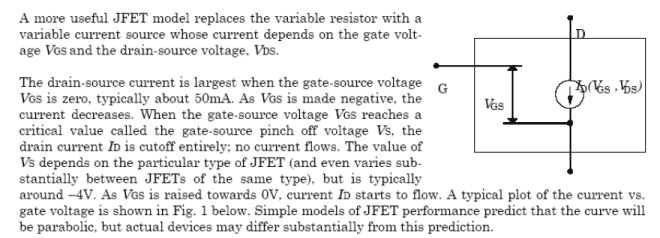• There is a convenient relationship between IDS and VGS.
• Beyond pinch-off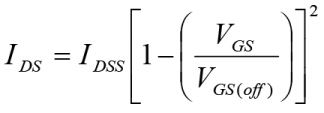• Where IDSS is drain current when VGS= 0 and VGS(off) is defined as –VP, that is gate-source voltage that just pinches off the channel.
• The pinch off voltage VP here is a +ve quantity because it was introduced through VDS(sat).
• VGS(off) however is negative, -VP.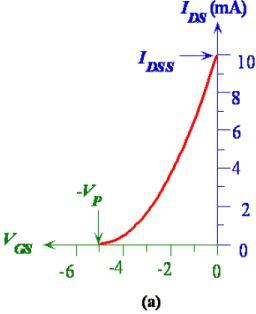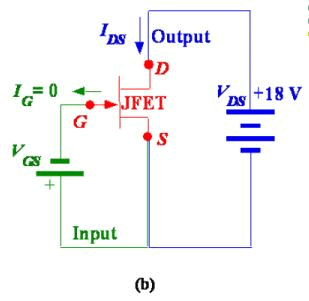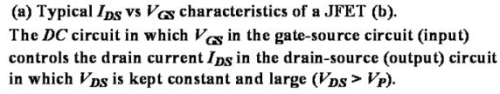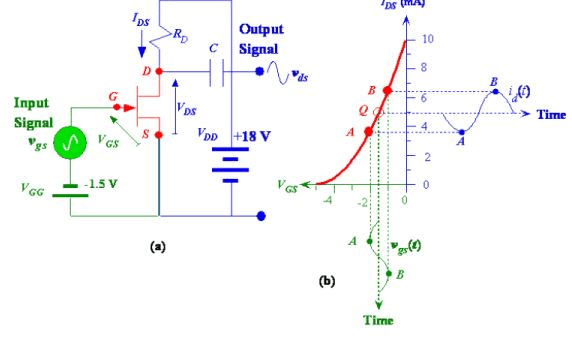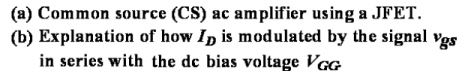I-V characteristics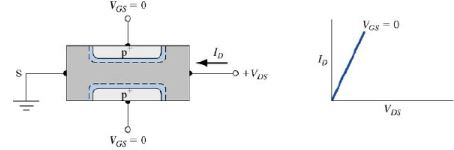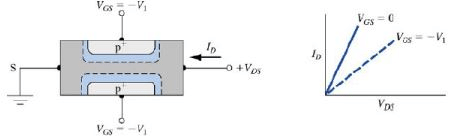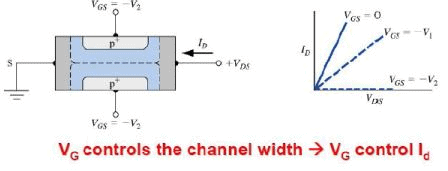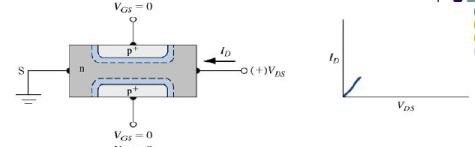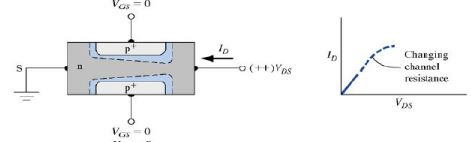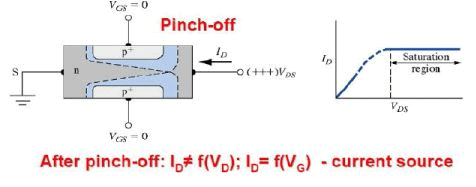JFET: I-V characteristics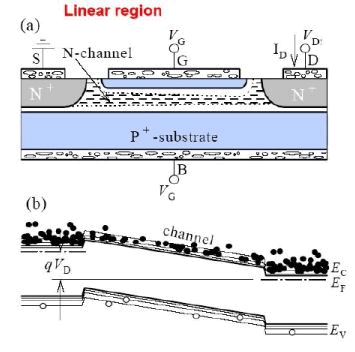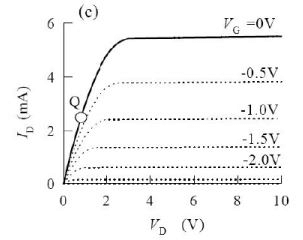The transconductance curve

• The process for plotting transconductance curve for a given JFET:
• Plot a point that corresponds to value of VGS(off).
• Plot a poit that corresponds to value of IDSS.
• Select 3 or more values of VGS between 0 V and VGS(off). For value of VGS, determine the corresponding value of ID from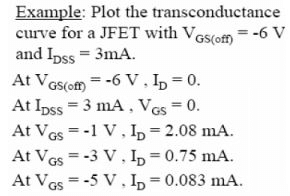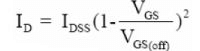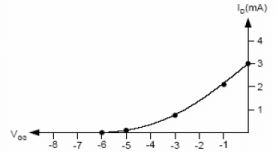• Plot the point from (3) and connect all the plotted point with a smooth curve.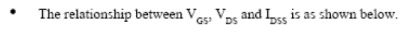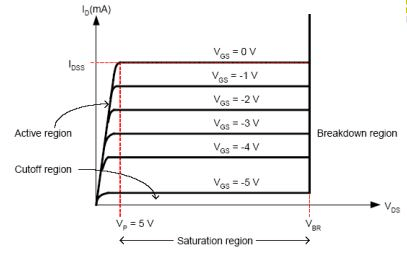JFET drain curves

JFET Biasing  Circuits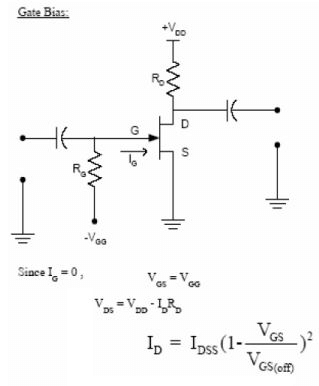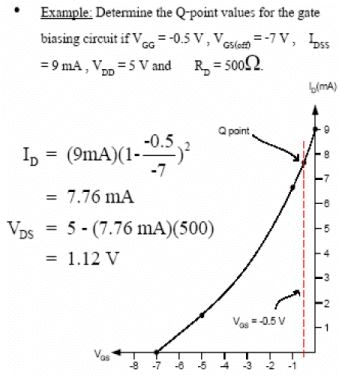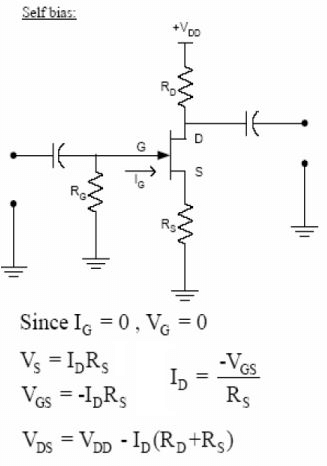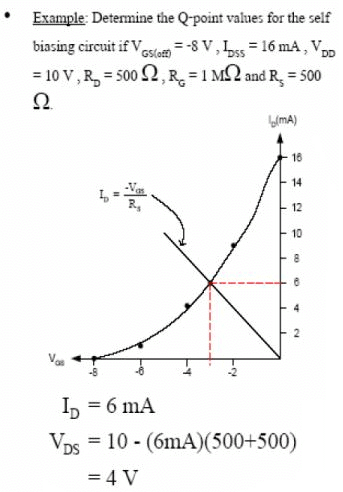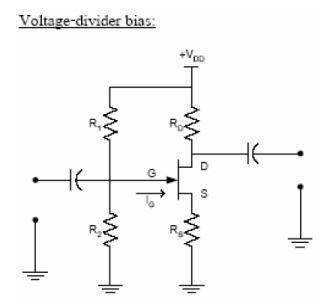The method used to plot the dc bias line for the voltage-divider bias is as follows:

1. Plot the transconductance curve for the specific JFET.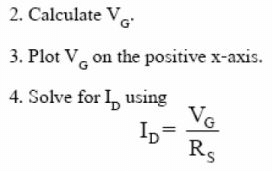5.  Plot ID found in (4) on the y-axis.

6. Extend the line to intersect the transconductance curve to obtain the Q- pomt values.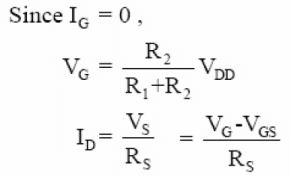Example: Plot the dc bias line for the voltagedrivers biasing circuit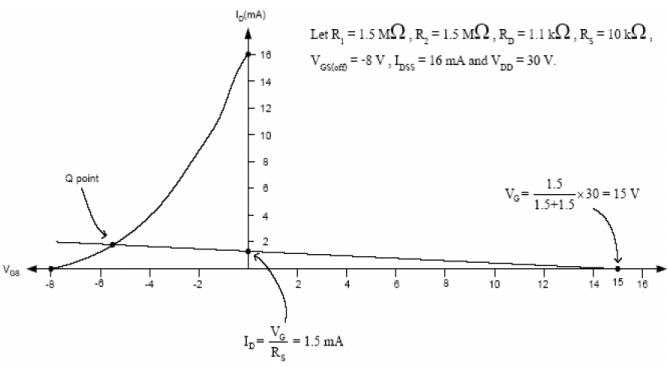The document Junction Field Effect Transistor - Semiconductor Devices, CSIR-NET Physical Sciences Notes | Study Physics for IIT JAM, UGC - NET, CSIR NET - Physics is a part of the Physics Course Physics for IIT JAM, UGC - NET, CSIR NET.
All you need of Physics at this link: Physics

## Physics for IIT JAM, UGC - NET, CSIR NET

159 docs
 Use Code STAYHOME200 and get INR 200 additional OFF

## Physics for IIT JAM, UGC - NET, CSIR NET

159 docs

Track your progress, build streaks, highlight & save important lessons and more!

,

,

,

,

,

,

,

,

,

,

,

,

,

,

,

,

,

,

,

,

,

,

,

,

,

,

,

,

,

,

;